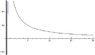# Convergence of a Series

• hpayandah

## Homework Statement

Ʃ$^{∞}_{n=1}$ $\frac{sin(1/n}{\sqrt{n}}$

## The Attempt at a Solution

lim$_{n→∞}$ $\frac{sin(1/n}{\sqrt{n}}$= lim$_{n→∞}$ $\frac{-2cos(1/n)}{n^{3/2}}$ with l'hospital rule = 0

since lim$_{n→∞}$=0 therefore the series is convergent

Do you think I did this right?

I can't imagine why you would use L'Hopital for that: sin(2/n) lies between -1 and 1 for all n while the numerator gets larger and larger. Of course, the limit is 0.

But that doesn't help you. $a_n$ going to 0 is a necessary condition for convergence of the series $\sum a_n$, it is not a sufficient condition.

If $a_n$ does NOT go to 0, then $\sum a_n$ does not converge but there exist series in which $a_n$ converges to 0 but the series $\sum a_n$ does not converge.

For example, $\sum 1/n$ clearly has 1/n going to 0 but the series does not converge.

I can't imagine why you would use L'Hopital for that: sin(2/n) lies between -1 and 1 for all n while the numerator gets larger and larger. Of course, the limit is 0.

But that doesn't help you. $a_n$ going to 0 is a necessary condition for convergence of the series $\sum a_n$, it is not a sufficient condition.

If $a_n$ does NOT go to 0, then $\sum a_n$ does not converge but there exist series in which $a_n$ converges to 0 but the series $\sum a_n$ does not converge.

For example, $\sum 1/n$ clearly has 1/n going to 0 but the series does not converge.

Consider doing a limit comparison test. What well known limit involving sine might help here?

Thank you for quick reply. Okay now I understand that the proof is not sufficient. Can I instead use the fact that you said -1 < sin(1/n) < 1 and the graph shows this:

Graph attached.

can I say since $\frac{sin(1/n}{\sqrt{n}}$ < $\frac{1}{\sqrt{n}}$ then use the p-series to show convergence or divergence.
or I can think of continuing what I was doing and prove that n^(3/2) converges then by definiton -2con(1/n) should also converge.

#### Attachments

•graph.jpg
4.1 KB · Views: 346
Thank you for quick reply. Okay now I understand that the proof is not sufficient. Can I instead use the fact that you said -1 < sin(1/n) < 1 and the graph shows this:

Graph attached.

can I say since $\frac{sin(1/n}{\sqrt{n}}$ < $\frac{1}{\sqrt{n}}$ then use the p-series to show convergence or divergence.
or I can think of continuing what I was doing and prove that n^(3/2) converges then by definiton -2con(1/n) should also converge.

You are doing way too much work. When n is large, 1/n is small and sin(1/n) is 1/n + O(1/n^2), so the nth term is 1/n^(3/2) + O(1/n^(5/2)). Convergence is then obvious.

RGV